•matlab
• 关于贝叶斯算法的一些资料 都是从网上找的 因为毕业设计要用到
• 机器学习——贝叶斯算法和朴素贝叶斯算法前言贝叶斯算法1、贝叶斯决策论算法示例 前言 先理解下贝叶斯算法要解决的问题： 正向概率： 假设袋子里面有N个白球，M个黑球，伸手进去摸一把，摸出黑球的概率有多大...
机器学习——分类算法之贝叶斯算法和朴素贝叶斯算法前言一、贝叶斯算法算法示例二、朴素贝叶斯分类器1、贝叶斯决策论2、多元正太分布参数的极大似然估计3、朴素贝叶斯分类器
前言
这部分的公式推导较多，涉及到了极大似然估计和贝叶斯估计，要看懂贝叶斯算法一定要明白极大似然估计。
其中贝叶斯算法基于贝叶斯公式，并不作为重点，重点在朴素贝叶斯算法，这里的公式推导很详细，一步步看下来一定会整明白的！！
一、贝叶斯算法
先理解下贝叶斯算法要解决的问题：

正向概率： 假设袋子里面有N个白球，M个黑球，伸手进去摸一把，摸出黑球的概率有多大？很简单：M/(N+M)

逆向概率： 如果事先并不知道袋子里黑白球的比例，而是闭着眼睛摸出一个（或好几个）球，观察这些取出来的球的颜色之后，那么就可以对此袋子里面的黑白球的比例作出什么样的推测
体会这两个概率问题的不同：正向概率问题已经事先知道概率分布，而逆向概率问题却不知道，那么贝叶斯算法就能派上用场了。
再举个计算题来体会贝叶斯算法(后面还会有一个)：

有一个人群，男人占60%，女人占40%，男人总是穿长裤，女人则有一半穿长裤一半穿裙子。
正向概率： 随机选取一个人，他（她）穿长裤子的概率和穿裙子的概率多大？
逆向概率： 迎面走来一个穿长裤的学生，只看得见他（她）穿的是长裤，而无法判断其性别，推断出其是女生的概率？
解：假设该群体有U个人，
穿长裤的男人的个数：U*P(男)*P(穿长裤|男)
其中，由题意可知P(男)=0.6，P(穿长裤|男)=1（男人全部穿长裤）
穿长裤的女人个数：U*P(女)*P(穿长裤|女)
-----------------------------------------------------------------------
那么对于正向概率问题——穿长裤的概率和穿裙子的概率分贝为：
P(穿长裤) = P(男)+P(女) * 0.5=0.6 + 0.4 * 0.5 = 0.8
P(穿裙子)=1-P(穿长裤)=P(女)*0.5= 0.2
------------------------------------------------------------------------
对于逆向问题——求该穿长裤的是女生的概率：
穿长裤总数：U*P(男)*P(穿长裤|男)+U*P(女)*P(穿长裤|女)
那么该穿长裤的是女生的概率P(女|穿长裤)=U*P(女)*P(穿长裤|女)/穿长裤总数=U*P(女)*P(穿长裤|女)/(U*P(男)*P(穿长裤|男)+U*P(女)*P(穿长裤|女))


注意到上面的逆向问题中，最后的结果是与总人数（U）无关的（分子分母约分），那么最后的结果可以写成
$P(女|穿长裤)=\frac{P(女)*P(穿长裤|女)}{P(男)*P(穿长裤|男)+P(女)*P(穿长裤|女)}\tag{1}$
化简下(1)式，观察(1)发现其分母就是穿长裤的概率P(穿长裤)，分子P(穿长裤，女)，那么此处就可以给出贝叶斯公式了：
$P(A|B)=\frac{P(B|A)P(A)}{P(B)}\tag{2}$
通俗的说直接求$P(A|B)$困难，那么就用上式转换下，换成好求的值（大白话）。
那么讲些官话：
贝叶斯定理是随机事件A和B的条件概率：
$P(A|B)=\frac{P(B|A)P(A)}{P(B)}$
其中P(A|B)是在 B 发生的情况下 A 发生的可能性。
成分定义如下：

P(A)是 A 的先验概率，之所以称为“先验”是因为它不考虑任何 B 方面的因素。
P(A|B)是已知 B 发生后 A 的条件概率，也由于得自 B 的取值而被称作 A 的后验概率。
P(B|A)是已知 A 发生后 B 的条件概率，也由于得自 A 的取值而被称作 B 的后验概率。
P(B)是 B 的先验概率，也作标淮化常量（normalizing constant）。

根据上面的定义，贝叶斯定理可表述为：
$后验概率 = (似然* 先验概率)/标淮化常量$
算法示例
这里借用其他博文的一个例子吧（不同任务算法不同，这里就不要脸的抄了）：
假设有两个各装了100个球的箱子，甲箱子中有70个红球，30个绿球，乙箱子中有30个红球，70个绿球。假设随机选择其中一个箱子，从中拿出一个球记下球色再放回原箱子，如此重复12次，记录得到8次红球，4次绿球。问题来了，你认为被选择的箱子是甲箱子的概率有多大？

刚开始选择甲乙两箱子的先验概率都是50%，因为是随机二选一（这是贝叶斯定理二选一的特殊形式）。即有：
P(甲) = 0.5， P(乙) = 1 - P(甲)；
这时在拿出一个球是红球的情况下，我们就应该根据这个信息来更新选择的是甲箱子的先验概率：
P(甲|红球1) = P(红球|甲) × P(甲) / (P(红球|甲) × P(甲) + (P(红球|乙) × P(乙)))
P(红球|甲)：甲箱子中拿到红球的概率
P(红球|乙)：乙箱子中拿到红球的概率
因此在出现一个红球的情况下，选择的是甲箱子的先验概率就可被修正为：
P(甲|红球1) = 0.7 × 0.5 / (0.7 × 0.5 + 0.3 × 0.5) = 0.7
即在出现一个红球之后，甲乙箱子被选中的先验概率就被修正为：
P(甲) = 0.7， P(乙) = 1 - P(甲) = 0.3
代码：
def bayesFunc(pIsBox1, pBox1, pBox2):
return (pIsBox1 * pBox1)/((pIsBox1 * pBox1) + (1 - pIsBox1) * pBox2)
def redGreenBallProblem():
pIsBox1 = 0.5
# consider 8 red ball
for i in range(1, 9):
pIsBox1 = bayesFunc(pIsBox1, 0.7, 0.3)
print ("拿到 %d 个球是红球是甲箱子的先验概率: %f" % (i, pIsBox1))
# consider 4 green ball
for i in range(1, 5):
pIsBox1 = bayesFunc(pIsBox1, 0.3, 0.7)
print ("拿到 %d 个球是绿球是甲箱子的先验概率: %f" % (i, pIsBox1))
redGreenBallProblem()

结果：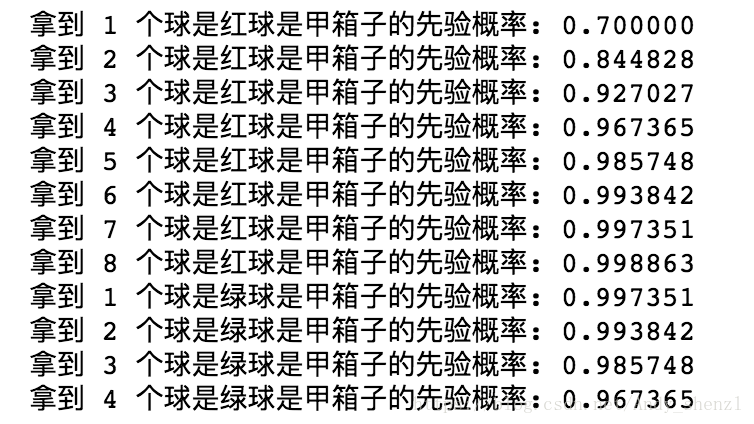还有一个贝叶斯手写字体识别示例
二、朴素贝叶斯分类器
1、贝叶斯决策论
先定义一些变量：

假设一个数据集有N种标记（标签），即$\gamma= \{c_{1},c_{2},...,c_{N}\}$
用$\lambda_{ij}$表示将一个真实标记为$c_{j}$的样本误分类为$c_{i}$所产生的损失
基于后验概率$P(c_{i}|x)$可获得将样本x分类为$c_{j}$所产生的期望损失，也是在样本x上的条件风险：$R(c_{i}|x) = \sum_{j=1}^{N}\lambda_{ij} P(c_{j}|x)\tag{3}$

贝叶斯算法的目的就是最小化(3)式损失、风险，即找到一个算法$h^{*}$，使得总体风险最小化：
$R(h)=E_{x}[R(h(x)|x)]\tag{4}$
若h能最小化每个样本$x_{i}$的条件风险$R(h(x)|x)$，则总体风险$R(h)$也将是最小的。这就产生了贝叶斯判定准则： 为了最小化总体风险，只需在每个样本上选择那个能使条件风险$R(c|x)$最小的类别标记，即：
$h^{*}(x)=\underset{c\in\gamma}{argmin}R(c|x)\tag{5}$
(一定要看懂(5)式，这里再强调下，$R(c|x)$是对单个样本来说所产生的条件风险，而算法$h^{*}(x)$的输入也是单个样本，也就是说，给算法$h^{*}(x)$传入一个样本，输出的是一个类别标记，而这个类别标记满足使得条件风险$R(c|x)$最小的要求)
此时，$h^{*}$被称为贝叶斯最优分类器，$R(h^{*})$称为贝叶斯风险,$1-R(h^{*})$反映了分类器所能达到的最好性能。(5)式只能最为求解贝叶斯最优分类器$h^{*}$的理论指导，并不能据此直接求解出$h^{*}$，所以要进一步推进！
下面进一步讨论条件风险::::::::::::::
从(3)式可知条件风险的计算公式为：
$R(c_{i}|x) = \sum_{j=1}^{N}\lambda P(c_{j}|x)$
若目标是最小化分类错误率，则误判损失$\lambda_{ij}$
对应为0/1损失，即：
$\lambda_{ij}=\left\{\begin{matrix} 0&,if i = j \\ 1& ,otherwise \end{matrix}\right.\tag{6}$
将(6)带入(3)式：
$R(c|x)=\lambda_{i1}*P(c_{1}|x)+\lambda_{i12}*P(c_{2}|x)+...+\lambda_{ii}*P(c_{i}|x)+\lambda_{ii+1}*P(c_{i+1}|x)+...+\lambda_{iN}*P(c_{N}|x)\\=1*P(c_{1}|x)+...+1*P(c_{i-1}|x)+0*P(c_{i}|x)+1*P(c_{i+1}|x)+...+1*P(c_{N}|x)\tag{7}$
(7)式中去掉乘0项，并由$P(c_{1}|x)+P(c_{2}|x)+...+P(c_{N}|x)=1$得：
$R(c|x)=P(c_{1}|x)+...+P(c_{i-1}|x)+P(c_{i+1}|x)+...+P(c_{N}|x)\\=1-P(c_{i}|x)\tag{8}$
那么由贝叶斯最优分类器的判定准则可知，最小化错误率的贝叶斯最优分类器为：
$h^{*}(x)=\underset{c\in\gamma}{argmin}R(c|x)=\underset {c\in\gamma}{argmin}(1-P(c|x))=\underset{c\in\gamma}{argmax}P(c|x)$
这样看来，最开始要根据风险最小找最优贝叶斯分类器，那么就等价于使得后验概率$P(c|x)$最大化。所以下面就开始求最大的后验概率$P(c|x)$。
2、多元正太分布参数的极大似然估计
如果关于极大似然估计不了解，可以看我的另一篇博文——极大似然估计和贝叶斯估计
至于这块为什么突然来了个“多元正太分布参数的极大似然估计”，因为后面的朴素贝叶斯算法会用到，所以这块也要推明白。
已知对数似然函数为：
$LL(\theta_{c})=\sum_{x\in D_{c}} log P(x|\theta_{c})\tag{9}$
注意上式中兵没有规定对数函数的底数是多少，但是只要保证这个对数函数是单调递增的就可以，所以在这里取自然常数e，那么此时的对数似然函数可以写成：
$LL(\theta_{c})=\sum_{x\in D_{c}} ln P(x|\theta_{c})\tag{10}$
这里已经假设数据集服从多元正态分布的，即：
$P(x|\theta_{c})=P(x|c)\sim \mathbb{N}(\mu_{c},\sigma_{c}^{2})\tag{11}$
所以有
$P(x|\theta_{c})=\frac{1}{ \sqrt {(2\pi)^{d}|\Sigma_{c}|}}exp[-\frac{1}{2}(x-\mu_{c})^{T}\Sigma_{c}^{-1}(x-\mu_{c})]\tag{12}$
(12)式不太好理解，这里解释一下。先看整体是正态分布的形式，而且这是多元函数的正太分布；再分别看：其中d表示x的维数，$\Sigma_{c}=\sigma_{c}^{2}$为对称正定协方差矩阵，$|\Sigma_{c}|$表示$\Sigma_{c}$的行列式。
将式(12)带入（10）中：
$LL(\theta_{c})=\sum_{x\in D_{c}}ln[\frac{1}{ \sqrt {(2\pi)^{d}|\Sigma_{c}|}}exp(-\frac{1}{2}(x-\mu_{c})^{T}\Sigma_{c}^{-1}(x-\mu_{c}))]\tag{13}$
令$|D_{c}|=N$(表示属于c类别的集合的样本总数)，则对数似然函数可化为：
$LL(\theta_{c})=\sum_{i=1}^{N}ln[\frac{1}{ \sqrt {(2\pi)^{d}|\Sigma_{c}|}}exp(-\frac{1}{2}(x_{i}-\mu_{c})^{T}\Sigma_{c}^{-1}(x_{i}-\mu_{c}))]\\=\sum_{i=1}^{N}ln[\frac{1}{ \sqrt {(2\pi)^{d}}}\frac{1}{\sqrt{|\Sigma_{c}|}}exp(-\frac{1}{2}(x_{i}-\mu_{c})^{T}\Sigma_{c}^{-1}(x_{i}-\mu_{c}))]\\=\sum_{i=1}^{N}[ln\frac{1}{ \sqrt {(2\pi)^{d}}}+ln\frac{1}{\sqrt{|\Sigma_{c}|}}+ln[exp(-\frac{1}{2}(x_{i}-\mu_{c})^{T}\Sigma_{c}^{-1}(x_{i}-\mu_{c}))]]\\=\sum_{i=1}^{N}[-\frac{d}{2}ln(2\pi)-\frac{1}{2}ln|\Sigma_{c}|-\frac{1}{2}((x_{i}-\mu_{c})^{T}\Sigma_{c}^{-1}(x_{i}-\mu_{c}))]\\=-\frac{Nd}{2}ln(2\pi)-\frac{N}{2}ln|\Sigma_{c}|-\frac{1}{2}\sum_{i=1}^{N}(x_{i}-\mu_{c})^{T}\Sigma_{c}^{-1}(x_{i}-\mu_{c})\tag{14}$
这里的参数$\widehat\theta$就是指$\mu_c和\Sigma_{c}$，所以下面分别求导数：
$\frac{\partial LL(\theta_{c})}{\partial \mu _{c}}=\frac{\partial}{\partial \mu_{c}}[-\frac{Nd}{2}ln(2\pi)-\frac{N}{2}ln|\Sigma_{c}|-\frac{1}{2}\sum_{i=1}^{N}(x_{i}-\mu_{c})^{T}\Sigma_{c}^{-1}(x_{i}-\mu_{c})]\\=\frac{\partial}{\partial \mu_{c}}[-\frac{1}{2}\sum_{i=1}^{N}(x_{i}-\mu_{c})^{T}\Sigma_{c}^{-1}(x_{i}-\mu_{c})]\\=-\frac{1}{2}\sum_{i=1}^{N}\frac{\partial}{\partial \mu_{c}}[(x_{i}-\mu_{c})^{T}\Sigma_{c}^{-1}(x_{i}-\mu_{c})]\\=-\frac{1}{2}\sum_{i=1}^{N}\frac{\partial}{\partial \mu_{c}}[(x_{i}^{T}-\mu_{c}^{T})\Sigma_{c}^{-1}(x_{i}-\mu_{c})]\\=-\frac{1}{2}\sum_{i=1}^{N}\frac{\partial}{\partial \mu_{c}}[(x_{i}^{T}-\mu_{c}^{T})(\Sigma_{c}^{-1}x_{i}-\Sigma_{c}^{-1}\mu_{c})]\\=-\frac{1}{2}\sum_{i=1}^{N}\frac{\partial}{\partial \mu_{c}}[x_{i}^{T}\Sigma_{c}^{-1T}x_{i}-x_{i}^{T}\Sigma_{c}^{-1}\mu_{c}-\mu_{c}^{T}\Sigma_{c}^{-1}x_{i}+\mu_{c}^{T}\Sigma_{c}^{-1}\mu_{c}]\tag{15}$
由于$x_{i}^{T}\Sigma_{c}^{-1}\mu_{c}$的计算结果为标量，并且$\Sigma_{c}$为实对称矩阵，所以：$x_{i}^{T}\Sigma_{c}^{-1}\mu_{c}=(x_{i}^{T}\Sigma_{c}^{-1}\mu_{c})^{T}=x_{i}^{T}(\Sigma_{c}^{-1})^{T}\mu_{c}=x_{i}^{T}(\Sigma_{c}^{T})^{-1}\mu_{c}=\mu_{c}^{T}\Sigma_{c}^{-1}x_{i}\tag{16}$
所以式(15)可以化简如下：
$\frac{\partial LL(\theta_{c})}{\partial \mu _{c}}=-\frac{1}{2}\sum_{i=1}^{N}\frac{\partial}{\partial \mu_{c}}[x_{i}^{T}\Sigma_{c}^{-1}x_{i}-2x_{i}^{T}\Sigma_{c}^{-1}\mu_{c}+\mu_{c}^{T}\Sigma_{c}^{-1}\mu_{c}]\\=-\frac{1}{2}\sum_{i=1}^{N}[0-(2x_{i}^{T}\Sigma_{c}^{-1})^{T}+(\Sigma_{c}^{-1}+(\Sigma_{c}^{-1})^{T})\mu_{c}]\\=-\frac{1}{2}\sum_{i=1}^{N}[-(2(\Sigma_{c}^{-1})^{T}x_{i})+(\Sigma _{c}^{-1}+(\Sigma _{c}^{-1})^{T})\mu_{c}]\\=-\frac{1}{2}\sum_{i=1}^{N}[-(2\Sigma_{c}^{-1}x_{i})+2\Sigma_{c}^{-1}\mu_{c}]\tag{17}\\=\sum_{i=1}^{N}\Sigma_{c}^{-1}x_{i}-N\Sigma_{c}^{-1}\mu_{c}$
令上式等于0，可得：
$\frac{\partial LL(\theta_{c})}{\partial \mu _{c}}=\sum_{i=1}^{N}\Sigma_{c}^{-1}x_{i}-N\Sigma_{c}^{-1}\mu_{c}=0\tag{18}$
即：$N\Sigma_{c}^{-1}\mu_{c}=\sum_{i=1}^{N}\Sigma_{c}^{-1}x_{i}=\Sigma_{c}^{-1}\sum_{i=1}^{N}x_{i}\tag{19}$
上式两边同乘$\Sigma_{c}$，得：$N\mu_{c}=\sum_{i=1}^{N}x_{i}\tag{20}$
那么最后可得$\mu_{c}$:
$\widehat\mu_{c}=\frac{1}{N}\sum_{i=1}^{N}x_{i}\tag{21}$
下面开始求$\Sigma_{c}$:
$\frac{\partial LL( \theta _{c})}{\partial \Sigma_{c}}=\frac{\partial}{\partial \Sigma_{c}}[-\frac{Nd}{2}ln(2\pi)-\frac{N}{2}ln|\Sigma_{c}|-\frac{1}{2}\sum_{i=1}^{N}(x_{i}-\mu_{c})^{T}\Sigma_{c}^{-1}(x_{i}-\mu_{c})]\\=\frac{\partial}{\partial \Sigma_{c}}[-\frac{N}{2}ln|\Sigma_{c}|-\frac{1}{2}\sum_{i=1}^{N}(x_{i}-\mu_{c})^{T}\Sigma_{c}^{-1}(x_{i}-\mu_{c})]\\=-\frac{N}{2}\frac{\partial}{\partial \Sigma_{c}}(ln|\Sigma_{c}|)-\frac{1}{2}\sum_{i=1}^{N}\frac{\partial}{\partial \Sigma_{c}}[(x_{i}-\mu_{c})^{T}\Sigma_{c}^{-1}(x_{i}-\mu_{c})]\tag{22}$
上式涉及到矩阵求偏导，由矩阵微分公式$\frac{\partial |X|}{\partial X}=|X|(X^{-1})^{T},\frac{\partial a^{T}X^{-1}b}{\partial X}=-X^{-T}ab^{T}X^{-T}$可得：
$\frac{\partial LL( \theta _{c})}{\partial \Sigma_{c}}=-\frac{N}{2}\frac{1}{| \Sigma_{c}|}| \Sigma_{c}|( \Sigma_{c}^{-1})^{T}-\frac{1}{2}\sum_{i=1}^{N}[-\Sigma_{c}^{-T}(x_{i}-\mu_{c})(x_{i}-\mu_{c})^{T}\Sigma_{c}^{-T}]\\=-\frac{N}{2}(\Sigma_{c}^{-1})^{T}-\frac{1}{2}\sum_{i=1}^{N}[-\Sigma_{c}^{-T}(x_{i}-\mu_{c})(x_{i}-\mu_{c})^{T}\Sigma_{c}^{-T}]\\=-\frac{N}{2}\Sigma_{c}^{-1}+\frac{1}{2}\sum_{i=1}^{N}[\Sigma_{c}^{-1}(x_{i}-\mu_{c})(x_{i}-\mu_{c})^{T}\Sigma_{c}^{-1}]\tag{23}$
令上式偏导数等于0，可得：
$\frac{\partial LL( \theta _{c})}{\partial \Sigma_{c}}=-\frac{N}{2}\Sigma_{c}^{-1}+\frac{1}{2}\sum_{i=1}^{N}[\Sigma_{c}^{-1}(x_{i}-\mu_{c})(x_{i}-\mu_{c})^{T}\Sigma_{c}^{-1}]=0\tag{24}$
下面开始化简式(24)，由(24)式可知：
$N\Sigma_{c}^{-1}=\sum_{i=1}^{N}[\Sigma_{c}^{-1}(x_{i}-\mu_{c})(x_{i}-\mu_{c})^{T}\Sigma_{c}^{-1}]\tag{25}$
将等式右边的$\Sigma_{c}^{-1}$提出来：
$N\Sigma_{c}^{-1}=\Sigma_{c}^{-1}[\sum_{i=1}^{N}(x_{i}-\mu_{c})(x_{i}-\mu_{c})^{T}]\Sigma_{c}^{-1}\tag{26}$
上式左右两边同乘$\Sigma_{c}$,得：
$N=\Sigma_{c}^{-1}[\sum_{i=1}^{N}(x_{i}-\mu_{c})(x_{i}-\mu_{c})^{T}]\tag{27}$
那么最终得到$\Sigma_{c}$为：
$\widehat\Sigma_{c}=\frac{1}{N}\sum_{i=1}^{N}(x_{i}-\mu_{c})(x_{i}-\mu_{c})^{T}\tag{28}$
3、朴素贝叶斯分类器
已知最小化分类错误率的贝叶斯最优分类器为：
$h^{*}(x)=\underset{c\in\gamma}{argmax}P(c|x)\tag{29}$
又由贝叶斯定理可知：
$P(c|x)=\frac{P(x,c)}{P(x)}=\frac{P(c)P(x|c)}{P(x)}\tag{30}$
把式(30)带入到（29)中
$h^{*}(x)= \underset{c\in\gamma}{argmax}\frac{P(c)P(x|c)}{P(x)}= \underset{c\in\gamma}{argmax}P(c)P(x|c)\tag{31}$
这里需要明白，为什么上式中的分母P(x)可以不计呢？还是回到贝叶斯判定准则，即找到使风险最小的标记c，那么这里的P(x)的分布是与标记c无关的，在变动c的时候P(x)是恒定不变的，所以可以去掉。
式（31）是普通贝叶斯最优分类器的表达式，那么朴素贝叶斯分类器就是在此基础上加上一个属性条件独立性假设，这也是所谓的“朴素”体现所在.
下面开始推导朴素贝叶斯分类器：
已知属性条件独立性假设为：
$P(x|c)=P(x_{1},x_{2},...,x_{d}|c)=\prod_{i=1}^{d}P(x_{i}|c)\tag{32}$
所以：
$h^{*}(x)=\underset{c\in \gamma}{argmax}P(c)\prod_{i=1}^{d}P(x_{i}|c)\tag{33}$
式(33)即为朴素贝叶斯分类器表达式。

现在解释下式(32)，根据概率论的知识可以知道，式(32)表达了$x_{1},x_{2},...,x_{d}$是相互独立的、相互不相关的，而这显然是不符合实际情形的，因为任何一个物品属性之间多多少少都应该有或多或少的关系，并不能说完全独立、完全不相关的。那么为什么要做属性之间相互独立的这种很强的假设呢？目的就是为了简便计算，也就是牺牲准确率，来换取效率。那么它是怎么简便计算的呢？下面举个简单地例子来说明：
假设某个数据集只有两个属性$x_{1},x_{2}$，其中$x_{1}=(a,b,c),x_{2}=(d,e,f)$，其对应的类别标记为$c = (1,2,3)$，现在想学习一个分类器，要把所有的参数都学习出来。这里所谓的所有的参数都学习出来，就是指各种属性取值前提下的联合概率，一共有27种联合概率（333）。现在有两种计算方案，其一是假设属性之间不独立，其二是假设属性之间是独立的。如果不独立的话就需要计算27次，而假设独立的前提下18次。（这块需要点概率论基础，看不懂的可以在下面评论，但是不懂也罢，只需要知道朴素贝叶斯可以降低计算量就可以了）
下面推导朴素贝叶斯的参数估计，怎样求先验概率$P(c)$和似然概率$P(x_{i}|c)$:
对于先验概率$P(c)$：
它表示的是样本空间中各类样本所占比例，根据大数定理，当训练集包含充足的独立同分布样本时，P（c）可通过各类样本出现的频率来进行估计，即：$P(c)=\frac{|D_{c}|}{|D|}\tag{34}$
其中，D表示训练集，|D|表示D中样本个数，$D_{c}$表示训练集中第c类样本组成的集合，$|D_{c}|$表示集合$D_{c}$中样本的个数

对于似然概率$P(x_{i}|c)$(这里以一元为例，多元正太分布的参数求解上面有)
这里需要分两种情况讨论：连续型和离散型。
若样本的第i个属性$x_{i}$取值为连续值，假设该属性的取值服从正态分布：
$P(x_{i}|c)\sim \mathbb{N}(x_{c,i},\sigma_{c,i}^{2})=>P(x_{i}|c)=\frac{1}{\sqrt{2\pi}\sigma_{c,i}}exp(-\frac{(x_{i}-\mu_{c,i})^{2}}{2\sigma_{c,i}^{2}})\tag{35}$
其中正态分布的参数可以用极大似然估计（区别于由大数定理得到的频率值）法推得：$\mu_{c,i}$和$\sigma_{c,i}^{2}$即为第c类样本在第i个属性上取值的均值和方差。
若样本的第i个属性$x_{i}$取值为离散值，同样根据极大似然估计法，用其频率值作为其概率值的估计值，即：
$P(x|c)=\frac{|D_{c,x_{i}}|}{|D_{c}|}\tag{36}$
其中，$D_{c,x_{i}}$表示$D_{c}$中第i 个属性上取值为$x_{i}$的样本组成的集合。
那么到此关于贝叶斯和朴素贝叶斯算法已经说完了，公式全部是手敲，这部分的算法公式又多，难免会存在遗漏、敲错等现象，还望指正！


展开全文机器学习
• 机器学习算法与自然语言处理推荐来源：...1.朴素贝叶斯算法朴素贝叶斯属于生成式模型(关于生成模型和判别式模型，主要还是在于是否需要求联合分布)，比较简单，你只需做...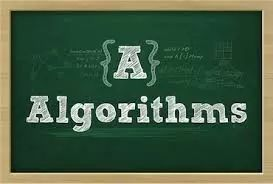机器学习算法与自然语言处理推荐 来源：http://www.cnblogs.com/pinard/p/6069267.html作者：刘建平Pinard【机器学习算法与自然语言处理导读】朴素贝叶斯算法是最经典的几个机器学习算法之一，本文对它的优点，缺点进行总结。1.  朴素贝叶斯算法朴素贝叶斯属于生成式模型(关于生成模型和判别式模型，主要还是在于是否需要求联合分布)，比较简单，你只需做一堆计数即可。如果注有条件独立性假设(一个比较严格的条件)，朴素贝叶斯分类器的收敛速度将快于判别模型，比如逻辑回归，所以你只需要较少的训练数据即可。即使NB条件独立假设不成立，NB分类器在实践中仍然表现的很出色。它的主要缺点是它不能学习特征间的相互作用，用mRMR中R来讲，就是特征冗余。引用一个比较经典的例子，比如，虽然你喜欢Brad Pitt和Tom Cruise的电影，但是它不能学习出你不喜欢他们在一起演的电影。2.  优点1. 朴素贝叶斯模型发源于古典数学理论，有着坚实的数学基础，以及稳定的分类效率；2. 对大数量训练和查询时具有较高的速度。即使使用超大规模的训练集，针对每个项目通常也只会有相对较少的特征数，并且对项目的训练和分类也仅仅是特征概率的数学运算而已；3. 对小规模的数据表现很好，能个处理多分类任务，适合增量式训练(即可以实时的对新增的样本进行训练)；4. 对缺失数据不太敏感，算法也比较简单，常用于文本分类；5. 朴素贝叶斯对结果解释容易理解。3.  缺点1. 需要计算先验概率；2. 分类决策存在错误率；3. 对输入数据的表达形式很敏感；4. 由于使用了样本属性独立性的假设，所以如果样本属性有关联时其效果不好。4.  应用领域1. 欺诈检测中使用较多；2. 一封电子邮件是否是垃圾邮件；3. 一篇文章应该分到科技、政治，还是体育类；4. 一段文字表达的是积极的情绪还是消极的情绪；5. 人脸识别。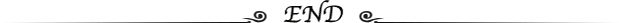推荐阅读：华为调研82位离职博士！任正非发电邮：反思人才流失根源漫话：如何给女朋友解释为什么双11无法修改收货地址2019 AI 国际顶级学术会议全在这里，请查收！展开全文• 朴素贝叶斯算法，贝叶斯分类算法，贝叶斯定理原理贝叶斯分类算法是统计学的一种分类方法，它是一类利用概率统计知识进行分类的算法。在许多场合，朴素贝叶斯(Naïve Bayes，NB)分类算法可以与决策树和神经网络分类...
朴素贝叶斯算法，贝叶斯分类算法，贝叶斯定理原理贝叶斯分类算法是统计学的一种分类方法，它是一类利用概率统计知识进行分类的算法。在许多场合，朴素贝叶斯(Naïve Bayes，NB)分类算法可以与决策树和神经网络分类算法相媲美，该算法能运用到大型数据库中，而且方法简单、分类准确率高、速度快。由于贝叶斯定理假设一个属性值对给定类的影响独立于其它属性的值，而此假设在实际情况中经常是不成立的，因此其分类准确率可能会下降。为此，就衍生出许多降低独立性假设的贝叶斯分类算法，如TAN(tree augmented Bayes network)算法。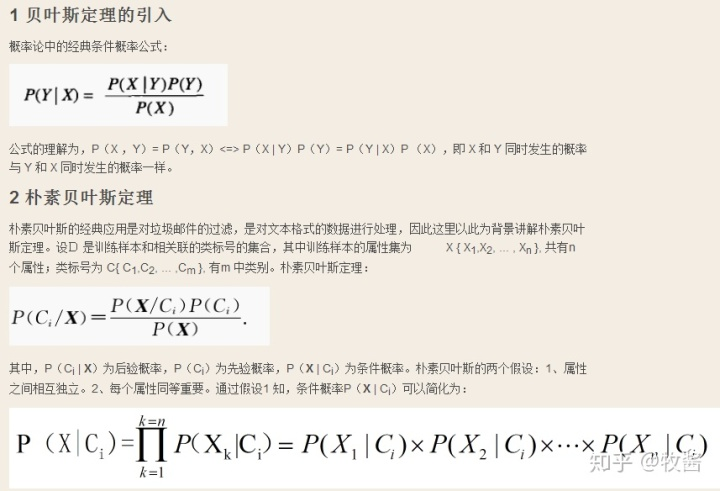朴素贝叶斯算法的核心思想：选择具有最高后验概率作为确定类别的指标。--------------------朴素贝叶斯算法设每个数据样本用一个n维特征向量来描述n个属性的值，即：X={x1，x2，…，xn}，假定有m个类，分别用C1, C2,…，Cm表示。给定一个未知的数据样本X（即没有类标号），若朴素贝叶斯分类法将未知的样本X分配给类Ci，则一定是P(Ci|X)>P(Cj|X) 1≤j≤m，j≠i根据贝叶斯定理由于P(X)对于所有类为常数，最大化后验概率P(Ci|X)可转化为最大化先验概率P(X|Ci)P(Ci)。如果训练数据集有许多属性和元组，计算P(X|Ci)的开销可能非常大，为此，通常假设各属性的取值互相独立，这样先验概率P(x1|Ci)，P(x2|Ci)，…，P(xn|Ci)可以从训练数据集求得。根据此方法，对一个未知类别的样本X，可以先分别计算出X属于每一个类别Ci的概率P(X|Ci)P(Ci)，然后选择其中概率最大的类别作为其类别。朴素贝叶斯算法成立的前提是各属性之间互相独立。当数据集满足这种独立性假设时,分类的准确度较高，否则可能较低。另外，该算法没有分类规则输出。　　　　在所有的机器学习分类算法中，朴素贝叶斯和其他绝大多数的分类算法都不同。对于大多数的分类算法，比如决策树,KNN,逻辑回归，支持向量机等，他们都是判别方法，也就是直接学习出特征输出Y和特征X之间的关系，要么是决策函数Y=f(X)Y=f(X),要么是条件分布P(Y|X)P(Y|X)。但是朴素贝叶斯却是生成方法，也就是直接找出特征输出Y和特征X的联合分布P(X,Y)P(X,Y),然后用P(Y|X)=P(X,Y)/P(X)P(Y|X)=P(X,Y)/P(X)得出。朴素贝叶斯很直观，计算量也不大，在很多领域有广泛的应用。1. 朴素贝叶斯相关的统计学知识　　　　在了解朴素贝叶斯的算法之前，我们需要对相关必须的统计学知识做一个回顾。　　　　贝叶斯学派很古老，但是从诞生到一百年前一直不是主流。主流是频率学派。频率学派的权威皮尔逊和费歇尔都对贝叶斯学派不屑一顾，但是贝叶斯学派硬是凭借在现代特定领域的出色应用表现为自己赢得了半壁江山。　　　　贝叶斯学派的思想可以概括为先验概率+数据=后验概率。也就是说我们在实际问题中需要得到的后验概率，可以通过先验概率和数据一起综合得到。数据大家好理解，被频率学派攻击的是先验概率，一般来说先验概率就是我们对于数据所在领域的历史经验，但是这个经验常常难以量化或者模型化，于是贝叶斯学派大胆的假设先验分布的模型，比如正态分布，beta分布等。这个假设一般没有特定的依据，因此一直被频率学派认为很荒谬。虽然难以从严密的数学逻辑里推出贝叶斯学派的逻辑，但是在很多实际应用中，贝叶斯理论很好用，比如垃圾邮件分类，文本分类。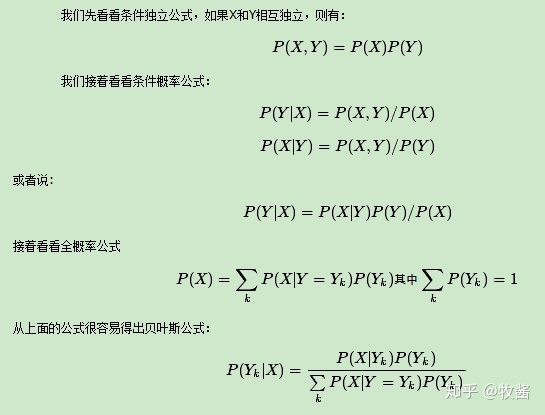2. 朴素贝叶斯的模型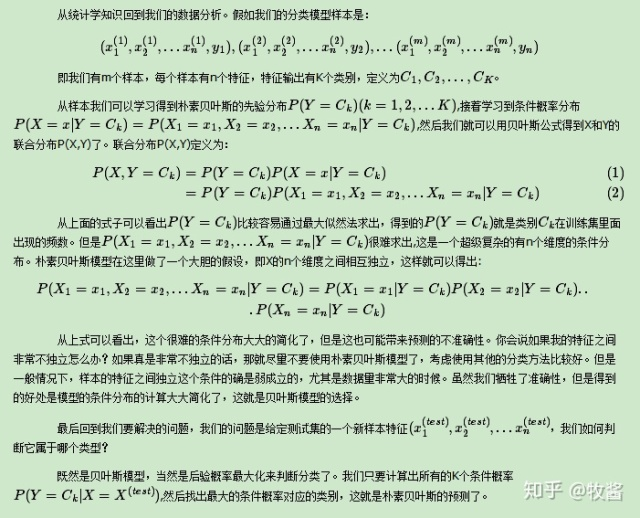3. 朴素贝叶斯的推断过程　　　　朴素贝叶斯的完整推断过程：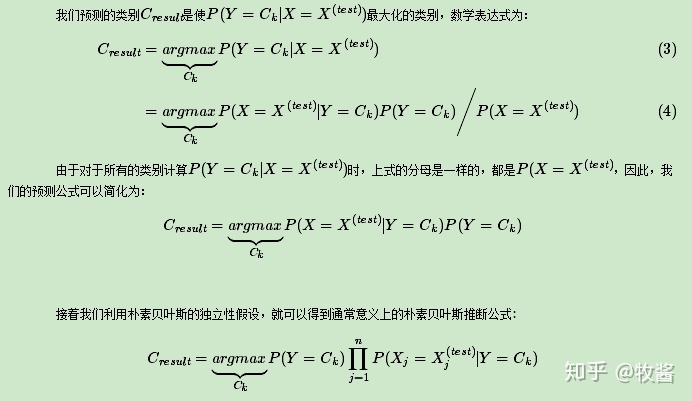4. 朴素贝叶斯的参数估计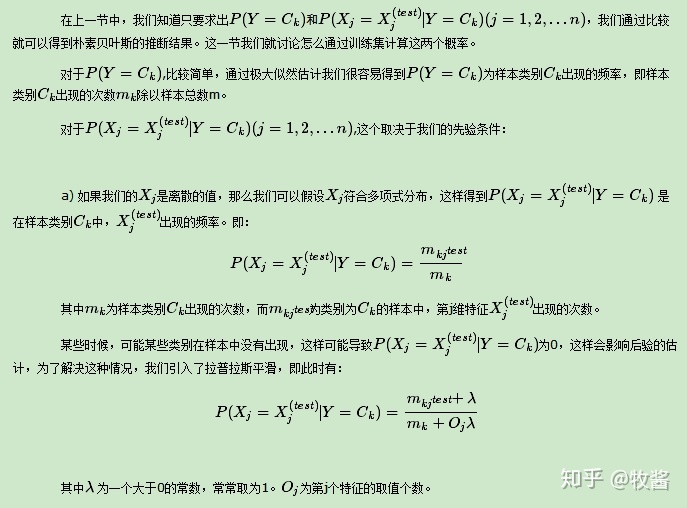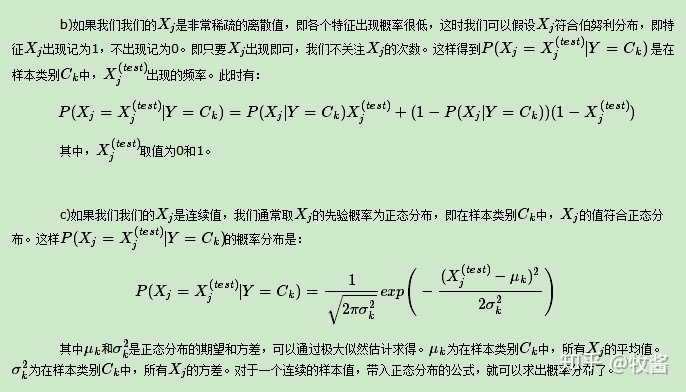5.  朴素贝叶斯算法过程　　　　我们假设训练集为m个样本n个维度，如下：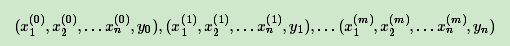共有K个特征输出类别，分别为C1,C2,...,CKC1,C2,...,CK,每个特征输出类别的样本个数为m1,m2,...,mKm1,m2,...,mK,在第k个类别中，如果是离散特征，则特征XjXj各个类别取值为mjlmjl。其中l取值为1,2,...Sj1,2,...Sj，SjSj为特征j不同的取值数。　　　　输出为实例X(test)X(test)的分类。　　　　算法流程如下：　　　　1) 如果没有Y的先验概率，则计算Y的K个先验概率：P(Y=Ck)=mk/mP(Y=Ck)=mk/m，否则P(Y=Ck)P(Y=Ck)为输入的先验概率。　　　　2) 分别计算第k个类别的第j维特征的第l个个取值条件概率：P(Xj=xjl|Y=Ck)P(Xj=xjl|Y=Ck)　　　　　　a)如果是离散值: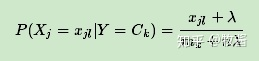λλ可以取值为1，或者其他大于0的数字。　　　　　　b)如果是稀疏二项离散值: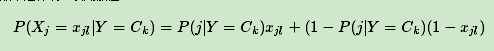此时ll只有两种取值。　　　　　　c)如果是连续值不需要计算各个l的取值概率，直接求正态分布的参数: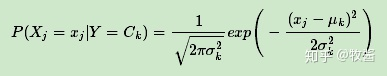需要求出μk和σ2kμk和σk2。 μkμk为在样本类别CkCk中，所有XjXj的平均值。σ2kσk2为在样本类别CkCk中，所有XjXj的方差。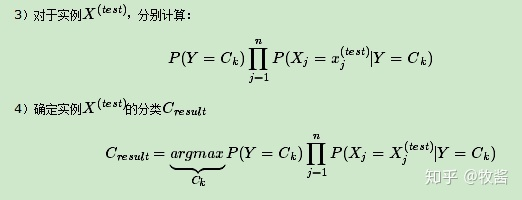从上面的计算可以看出，没有复杂的求导和矩阵运算，因此效率很高。6.  朴素贝叶斯算法小结　　　　朴素贝叶斯的主要优点有：　　　　1）朴素贝叶斯模型发源于古典数学理论，有稳定的分类效率。　　　　2）对小规模的数据表现很好，能个处理多分类任务，适合增量式训练，尤其是数据量超出内存时，我们可以一批批的去增量训练。　　　　3）对缺失数据不太敏感，算法也比较简单，常用于文本分类。　　　　朴素贝叶斯的主要缺点有：　　　　　　　1） 理论上，朴素贝叶斯模型与其他分类方法相比具有最小的误差率。但是实际上并非总是如此，这是因为朴素贝叶斯模型给定输出类别的情况下,假设属性之间相互独立，这个假设在实际应用中往往是不成立的，在属性个数比较多或者属性之间相关性较大时，分类效果不好。而在属性相关性较小时，朴素贝叶斯性能最为良好。对于这一点，有半朴素贝叶斯之类的算法通过考虑部分关联性适度改进。　　　　2）需要知道先验概率，且先验概率很多时候取决于假设，假设的模型可以有很多种，因此在某些时候会由于假设的先验模型的原因导致预测效果不佳。　　　　3）由于我们是通过先验和数据来决定后验的概率从而决定分类，所以分类决策存在一定的错误率。　　　　4）对输入数据的表达形式很敏感。
展开全文• ## 朴素贝叶斯算法

万次阅读 多人点赞 2019-07-07 22:32:22
贝叶斯算法概述 贝叶斯算法的提出： 贝叶斯定理 贝叶斯推导实例 男生、女生穿长裤 拼写纠错实例 贝叶斯算法概述 正向概率：假设袋子里面有N个白球，M个黑球，你伸手进去摸出黑球的概率...
本文参考唐宇迪的课程，博客https://www.zhoulujun.cn/html/theory/math/2017_0913_8050.html

目录

贝叶斯算法概述

贝叶斯算法的提出：

贝叶斯定理

贝叶斯推导实例

男生、女生穿长裤

拼写纠错实例

贝叶斯算法概述

正向概率：假设袋子里面有N个白球，M个黑球，你伸手进去摸出黑球的概率是多大？

逆向概率：如果我们事先并不知道袋子里面黑白球的比例，而是闭着眼睛摸出一个或者好几个球，观察这些取出来的球的颜色之后，那么我们可以就此对袋子里面的黑白球的比例作出什么样的预测呢？

关于上面的两个问题，正向概率我们很容易想到如何去解决，但是逆向概率，我们就不知道如何下手了，不过不要担心，贝叶斯就是为了解决“逆概率”而提出的。

贝叶斯算法的提出：

长久以来，人们对一件事情发生或不发生的概率，只有固定的0和1，即要么发生，要么不发生，从来不会去考虑某件事情发生的概率有多大，不发生的概率又是多大。而且概率虽然未知，但最起码是一个确定的值。比如如果问那时的人们一个问题：“有一个袋子，里面装着若干个白球和黑球，请问从袋子中取得白球的概率是多少？”他们会想都不用想，会立马告诉你，取出白球的概率就是1/2，要么取到白球，要么取不到白球，即θ只能有一个值，而且不论你取了多少次，取得白球的概率θ始终都是1/2，即不随观察结果X 的变化而变化。

这种频率派的观点长期统治着人们的观念，但是：假设我们有如下的7个球在A,B两个框中，如果我们随便取一个球，已知取到的球来自B框中，那么这个球是白球的概率是多少呢？或者问去除的球是白色，那么取自B框的概率是多少呢？这个问题不是很好解决，直到后来一个名叫Thomas Bayes的人物出现。

托马斯·贝叶斯Thomas Bayes（1702-1763）在世时，并不为当时的人们所熟知，很少发表论文或出版著作，与当时学术界的人沟通交流也很少，用现在的话来说，贝叶斯就是活生生一民间学术“屌丝”，可这个“屌丝”最终发表了一篇名为“An essay towards solving a problem in the doctrine of chances”，翻译过来则是：机遇理论中一个问题的解。你可能觉得我要说：这篇论文的发表随机产生轰动效应，从而奠定贝叶斯在学术史上的地位。回到上面的例子：“有一个袋子，里面装着若干个白球和黑球，请问从袋子中取得白球的概率θ是多少？”贝叶斯认为取得白球的概率是个不确定的值，因为其中含有机遇的成分。比如，一个朋友创业，你明明知道创业的结果就两种，即要么成功要么失败，但你依然会忍不住去估计他创业成功的几率有多大？你如果对他为人比较了解，而且有方法、思路清晰、有毅力、且能团结周围的人，你会不由自主的估计他创业成功的几率可能在80%以上。这种不同于最开始的“非黑即白、非0即1”的思考方式，便是贝叶斯式的思考方式。

继续深入讲解贝叶斯方法之前，先简单总结下频率派与贝叶斯派各自不同的思考方式：

频率派把需要推断的参数θ看做是固定的未知常数，即概率θ虽然是未知的，但最起码是确定的一个值，同时，样本X 是随机的，所以频率派重点研究样本空间，大部分的概率计算都是针对样本X 的分布；

而贝叶斯派的观点则截然相反，他们认为参数θ是随机变量，而样本X 是固定的，由于样本是固定的，所以他们重点研究的是参数θ的分布。

相对来说，频率派的观点容易理解，所以下文重点阐述贝叶斯派的观点。 贝叶斯派既然把θ看做是一个随机变量，所以要计算θ的分布，便得事先知道θ的无条件分布，即在有样本之前（或观察到X之前），θ有着怎样的分布呢？比如往台球桌上扔一个球，这个球落会落在何处呢？如果是不偏不倚的把球抛出去，那么此球落在台球桌上的任一位置都有着相同的机会，即球落在台球桌上某一位置的概率服从均匀分布。这种在实验之前定下的属于基本前提性质的分布称为先验分布，或者无条件分布。

至此，贝叶斯及贝叶斯派提出了一个思考问题的固定模式：

先验分布 π(θ)+ 样本信息χ⇒  后验分布π(θ|x)

上述思考模式意味着，新观察到的样本信息将修正人们以前对事物的认知。换言之，在得到新的样本信息之前，人们对的认知是先验分布 π(θ)，在得到新的样本信息后χ，人们对θ的认知为π(θ|x)。

而后验分布π(θ|x)一般也认为是在给定样本χ的情况下θ的条件分布，而使达到最大的值称为最大后θMD验估计，类似于经典统计学中的极大似然估计。

综合起来看，则好比是人类刚开始时对大自然只有少得可怜的先验知识，但随着不断是观察、实验获得更多的样本、结果，使得人们对自然界的规律摸得越来越透彻。所以，贝叶斯方法既符合人们日常生活的思考方式，也符合人们认识自然的规律，经过不断的发展，最终占据统计学领域的半壁江山，与经典统计学分庭抗礼。

此外，贝叶斯除了提出上述思考模式之外，还特别提出了举世闻名的贝叶斯定理。

贝叶斯定理

在引出贝叶斯定理之前，先学习几个定义：

边缘概率（又称先验概率）：某个事件发生的概率。边缘概率是这样得到的：在联合概率中，把最终结果中那些不需要的事件通过合并成它们的全概率，而消去它们（对离散随机变量用求和得全概率，对连续随机变量用积分得全概率），这称为边缘化（marginalization），比如A的边缘概率表示为P(A)，B的边缘概率表示为P(B)。

联合概率表示两个事件共同发生的概率。A与B的联合概率表示为P(A∩B)或者P(A,B)。

条件概率（又称后验概率）：事件A在另外一个事件B已经发生条件下的发生概率。条件概率表示为P(A|B)，读作“在B条件下A的概率”,。

接着，考虑一个问题：P(A|B)是在B发生的情况下A发生的可能性。

首先，事件B发生之前，我们对事件A的发生有一个基本的概率判断，称为A的先验概率，用P(A)表示；

其次，事件B发生之后，我们对事件A的发生概率重新评估，称为A的后验概率，用P(A|B)表示；

类似的，事件A发生之前，我们对事件B的发生有一个基本的概率判断，称为B的先验概率，用P(B)表示；

同样，事件A发生之后，我们对事件B的发生概率重新评估，称为B的后验概率，用P(B|A)表示。

贝叶斯定理便是基于下述贝叶斯公式：P(A|B)=P(B|A)P(A)/P(B)

上述公式的推导其实非常简单，就是从条件概率推出。

根据条件概率的定义，在事件B发生的条件下事件A发生的概率是

P(A|B)=P(A∩B)/P(B)

同样地，在事件A发生的条件下事件B发生的概率

P(B|A)=P(A∩B)/P(A)

整理与合并上述两个方程式，便可以得到：

P(A|B)P(B)=P(A∩B)=P(B|A)P(A)

接着，上式两边同除以P(B)，若P(B)是非零的，我们便可以得到贝叶斯定理的公式表达式：

P(A|B)=P(B|A)*P(A)/P(B)

P(A|B) 和 P(B|A) 之类的经常让人混淆，有人给出了理解的一个关键点，区分出规律和现象，就是将A看成“规律”，B看成“现象”，那么贝叶斯公式看成：《这的理解贝叶斯公式吗》、《又一个生活中的贝叶斯应用》也给出了几个通俗易懂的例子，感兴趣的同学可以去看看。

贝叶斯推断的含义

然后搜下，发现其实还有更好阐释，比如

对条件概率公式进行变形，可以得到形式：后验概率　＝　先验概率 ｘ 调整因子。

我们把P(A)称为"先验概率"（Prior probability），即在B事件发生之前，我们对A事件概率的一个判断。P(A|B)称为"后验概率"（Posterior probability），即在B事件发生之后，我们对A事件概率的重新评估。

P(B|A)/P(B)称为"可能性函数"（Likelyhood），这是一个调整因子，使得预估概率更接近真实概率。

所以，条件概率可以理解成下面的式子：

后验概率　＝　先验概率 ｘ 调整因子

这就是贝叶斯推断的含义。我们先预估一个"先验概率"，然后加入实验结果，看这个实验到底是增强还是削弱了"先验概率"，由此得到更接近事实的"后验概率"。

在这里，如果"可能性函数"P(B|A)/P(B)>1，意味着"先验概率"被增强，事件A的发生的可能性变大；如果"可能性函数"=1，意味着B事件无助于判断事件A的可能性；如果"可能性函数"<1，意味着"先验概率"被削弱，事件A的可能性变小。

最后，我们为什么需要贝叶斯呢？1. 现实世界本身就是不确定的，人类的观察能力是有局限性的。​ 2.​ 我们日常所观察到的只是事物表面上的结果，因此我们需要提供一个猜测。

贝叶斯推导实例

男生、女生穿长裤

一个学校的男生、女生比例为6：4，男生总是穿长裤，女生则一半穿长裤一半穿裙子。则正向概率问题是：随机选取一个学生，他（她）穿长裤的概率和穿裙子的概率是多大？逆向概率问题是：迎面走来一个穿长裤的学生，你只看得见他（她）穿的是否长裤，而无法确定他（她）的性别，你能够推断出他（她）是女生的概率是多大吗？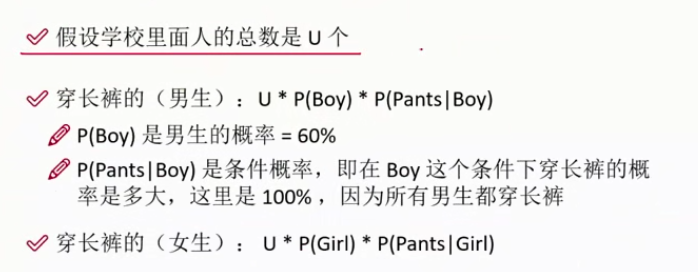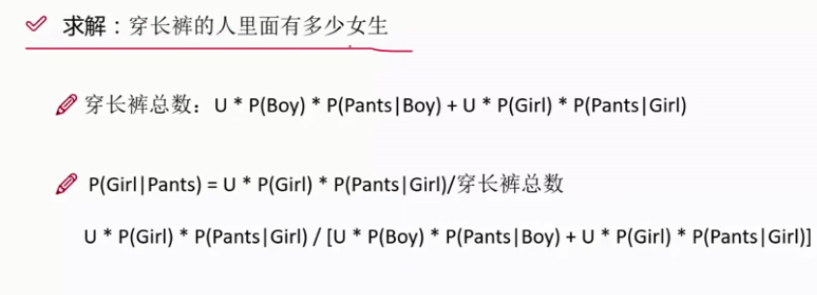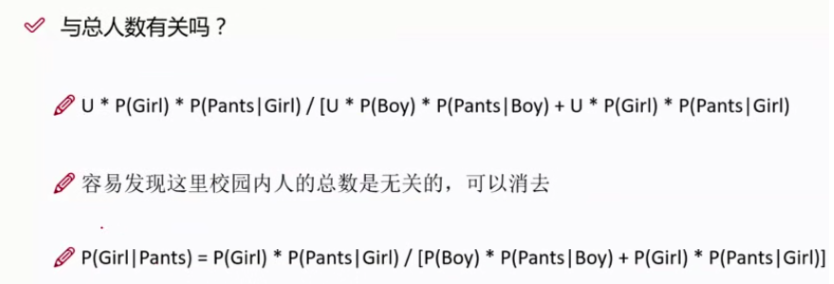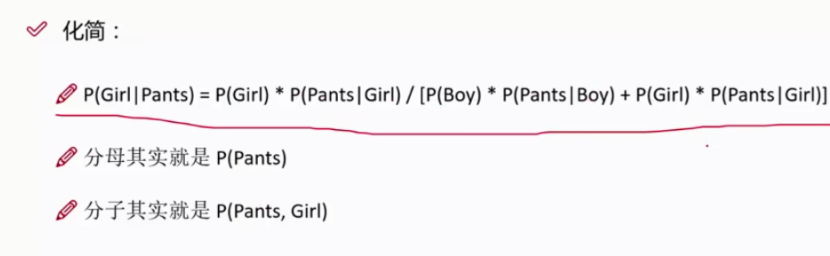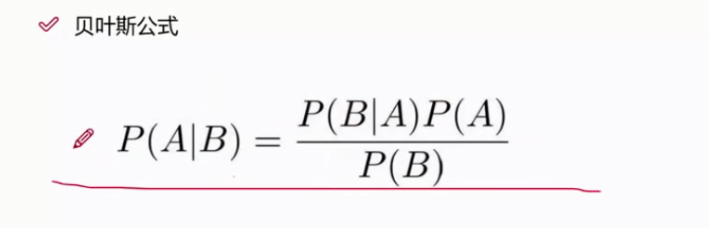拼写纠错实例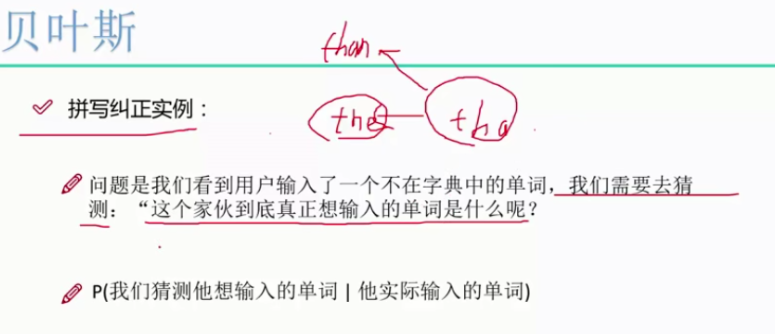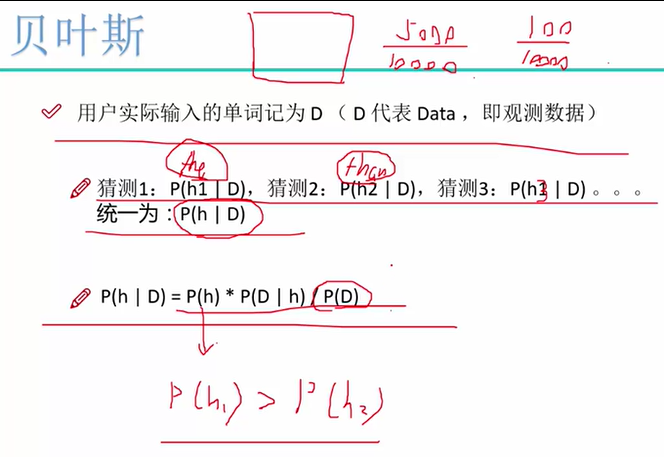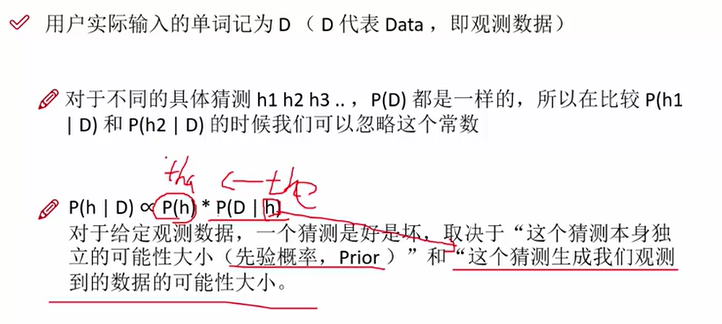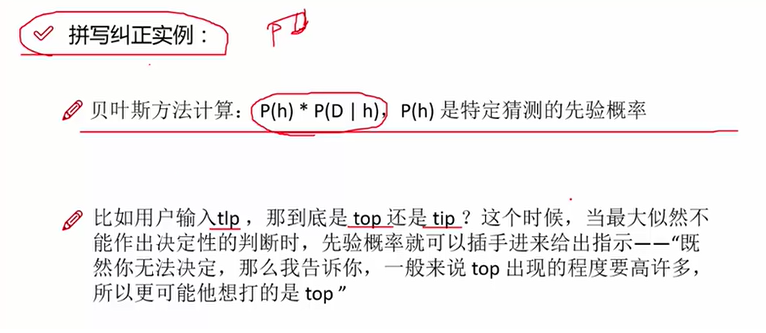展开全文• 机器学习朴素贝叶斯算法 朴素贝叶斯算法 (Naive Bayes Algorithm) Naive Bayes is basically used for text learning. Using this algorithm we trained machine from text. 朴素贝叶斯基本上用于文本学习。 使用此...机器学习 人工智能 深度学习 python
• 朴素贝叶斯算法;算法流程图;朴素贝叶斯算法原理;朴素贝叶斯算法原理;条件概率 表示事件B已经发生的前提下事件A发生的概率叫做事件B发生下事件A的条件概率其基本求解公式为 为了得出 我们不加证明的给出贝叶斯定理 ;...
• 今天把总结朴素贝叶斯算法（NaiveBayes）的学习心得。 Bayes是谁 Thomas Bayes，英国数学家。约1701年出生于伦敦，做过神甫。1742年成为英国皇家学会会员。1761年4月7日逝世。贝叶斯在数学方面主要研究概率论。他...算法 数据挖掘
• 朴素贝叶斯算法 Naive Bayes 算法流程图 朴素贝叶斯算法原理 朴素贝叶斯算法是分类算法中的一种朴素贝叶斯的思想基础是这样的对于给定的待分类项 求解在此项出现的条件下各个类别 出现的概率哪个 最大就把此待分类项...
• 今天抽空聊一聊机器学习中非常重要的一种算法——朴素贝叶斯(Naive Bayesian)。 先说说为什么叫做“朴素贝叶斯”，原因很简单：因为... 朴素贝叶斯算法的核心原理是：对于待分类样本，计算在此待分类样本出现的条件...
• YxImageV1.1 版 贝叶斯算法bug 贝叶斯算法bug 贝叶斯算法bug...